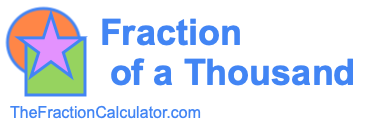Fraction of a Thousand CalculatorWelcome to the Fraction of a Thousand Calculator. This calculator performs two tasks for you. First, it calculates your fraction of a thousand and explains what it means. In addition, it illustrates what your fraction of a thousand looks like on a pie chart.

What is  /  of a thousand?

Here are some examples of what the Fraction of a Thousand Calculator can answer for you:

What is 1/3 of a thousand?

What is 1/4 of a thousand?

What is 3/4 of a thousand?

What is 4/5 of a thousand?